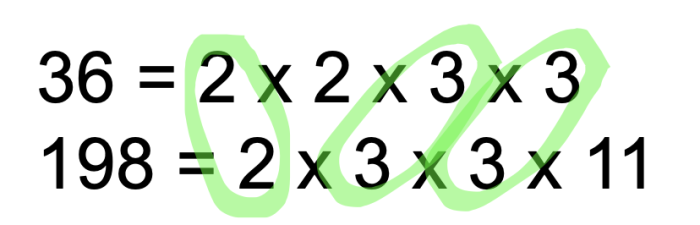# Find the HCF Using Prime Factors

In this worksheet, students will use prime factorisation to find the HCF of large numbers.Key stage:  KS 3

Curriculum topic:   Number

Curriculum subtopic:   Use Concepts and Vocabulary for All Numbers

Difficulty level:#### Worksheet Overview

In this activity, we will be finding the Highest Common Factor or the HCF for given values.

For smaller values we can do this by listing all of the factors for given numbers. However, this becomes an arduous task when we have larger values.

Instead we can do this by comparing the prime factors of the values given.

Example

Find the HCF of 36 and 198.

First, we start by writing 36 and 198 as products of their prime factors.

36 = 2 x 2 x 3 x 3

198 = 2 x 3 x 3 x 11

To find the HCF we multiply together the prime factors that appears in both lists.HCF of 36 and 198 = 2 x 3 x 3 = 18

Example 2

Find the HCF of 120 and 155.

First, we start by writing 120 and 155 as products of their prime factors.

120 = 2 x 2 x 2 x 3 x 5

155 = 5 x 31

To find the HCF we multiply together the prime factors that appear in both lists.

For both of these numbers 5 is the only value that appears in both lists.

HCF of 120 and 155 is 5.

Let's have a go at some questions now.

### What is EdPlace?

We're your National Curriculum aligned online education content provider helping each child succeed in English, maths and science from year 1 to GCSE. With an EdPlace account you’ll be able to track and measure progress, helping each child achieve their best. We build confidence and attainment by personalising each child’s learning at a level that suits them.

Get started